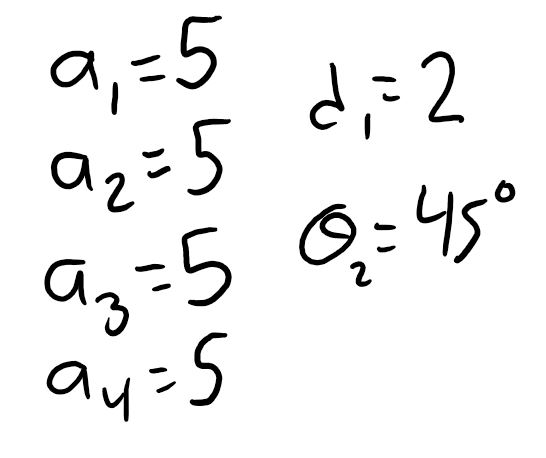Example Question 2:  Shown here is a kinematic diagram that is unlabeled.  Draw frames on this diagram according to the Denavit-Hartenberg rules, and label the joint variables and link lengths.
Example Question 3:  Shown here is a labeled kinematic diagram.  Find the complete homogeneous transformation matrix (HTM) from the base-frame to the end-effector frame by first finding the rotation matrices and the displacement vectors, then assembling them into the HTM.
Example Question 4 (Graduates Only):  Solve Example Question 3 using the Denavit-Hartenberg method.
Example Question 5: Shown here is a kinematic diagram along with its homogeneous transformation matrix.  Suppose that the joint variables and the link lengths have the values shown.  What is the (X,Y,Z) position of the end-effector in the base-frame?
Example Question 6:  Here I have drawn a frame that represents the base-frame of a manipulator.  Also, I have given the homogeneous transformation matrix for a manipulator that is not shown.  When the joint variables and link lengths are as given, what is the location and orientation of the end-effector frame?  Give your answer by drawing the end-effector frame on the picture.
Example Question 7: Shown here is the kinematic diagram of a 2-degree-of-freedom manipulator.  Find the inverse kinematics equations.
Example Question 8 (Graduates Only): Shown here is the kinematic diagram of a 3-degree-of-freedom manipulator.  Find the inverse kinematics equations.
Example Question 1: Shown here is a picture of an actual robot manipulator.  Draw a kinematic diagram of this manipulator.  Also write down which standard type of manipulator this is.
Congratulations!  You've made it all the way through our first unit: Kinematics.  Before we move on, you will need to take a test to make sure that all this information has sunk in.  Here are some example problems like the ones you will see on the test in class.  On our review day (the class day before the test), I'll go through these problems live on the board.  So, it's a good idea to try to solve the problems on your own before coming to class.
Example Question 9: Define or describe, in words and pictures, the four Denavit-Hartenberg rules for assigning coordinate frames.
Example Question 10 (Graduates Only): Define or describe, in words and pictures, the four Denavit-Hartenberg parameters.Example Question 11: Shown here is a kinematic diagram drawn by someone else.  There are 4 errors in this diagram.  Find the 4 errors and write (in words) what is wrong.Example Question 12: Shown here is a rotation matrix that was calculated by someone else.  Without knowing the manipulator or the kinematic diagram that this rotation matrix came from, you can know that this rotation matrix is wrong.  Explain how you know this.Robotics 1
Checkpoint 1: Kinematics
Watch the solution to Exam 1 2017: